Function Repository Resource:

# TheilSenLine

Fit a line through a set of points using the Theil–Sen estimator

Contributed by: Rauan Kaldybayev
 ResourceFunction["TheilSenLine"][data] uses the Theil–Sen estimator to fit a line through a set of points represented by data. ResourceFunction["TheilSenLine"][xlist,ylist] uses the Theil–Sen estimator to fit a line through a set of points, where xlist and ylist respectively give the x and y coordinates of the points. ResourceFunction["TheilSenLine"][…,x] returns an equation for the Theil-Sen line in terms of the variable x.

## Details

ResourceFunction["TheilSenLine"][data,x] or ResourceFunction["TheilSenLine"][xlist,ylist,x] returns the equation of a line mx+b, where x is the predictor of the model and can be any symbol.
ResourceFunction["TheilSenLine"][data] or ResourceFunction["TheilSenLine"][xlist,ylist] returns {m,b}.

## Examples

### Basic Examples (2)

Fit a line through the points (0,1),(1,1),(2.5,3) using the Theil–Sen estimator:

 In:=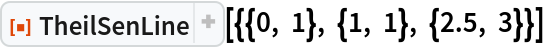Out=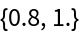The output is of the form {m,b}, which represents a line fit of the form y=mx+b. Compare the fitted line to the original points:

 In:=Out=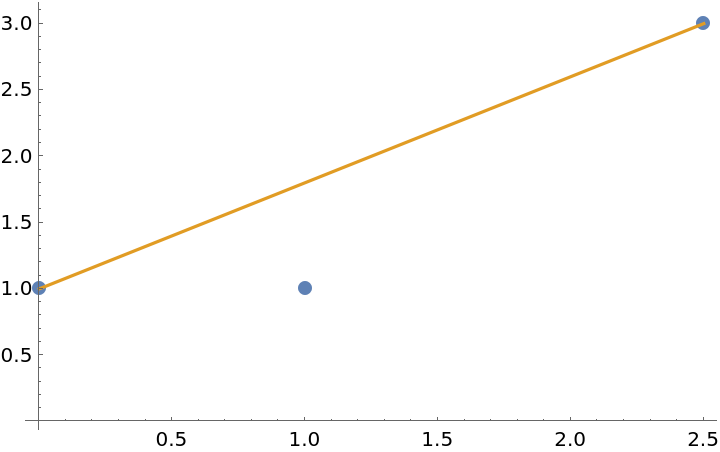### Scope (2)

Supply data given in the form of separate lists for the x and y coordinates:

 In:=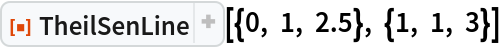Out=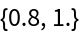Return a formula for the model by passing the predictor as an additional argument:

 In:=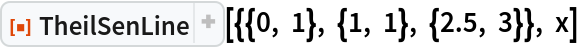Out=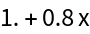## Publisher

Wolfram Summer Camp

## Version History

• 1.1.0 – 27 February 2023
• 1.0.1 – 10 February 2023
• 1.0.0 – 20 July 2021

The code for this function is based on a piece of code written by the StackExchange user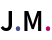in answering the question "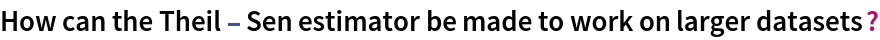" on Mathematica Stack Exchange.